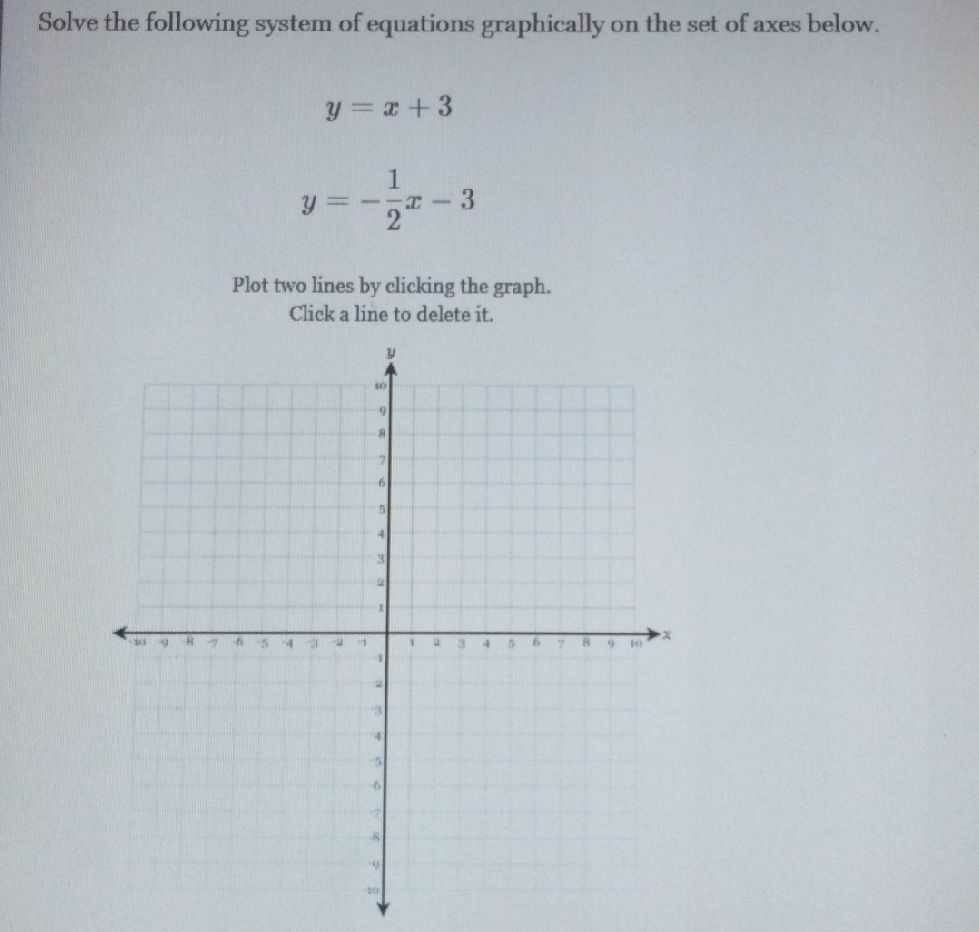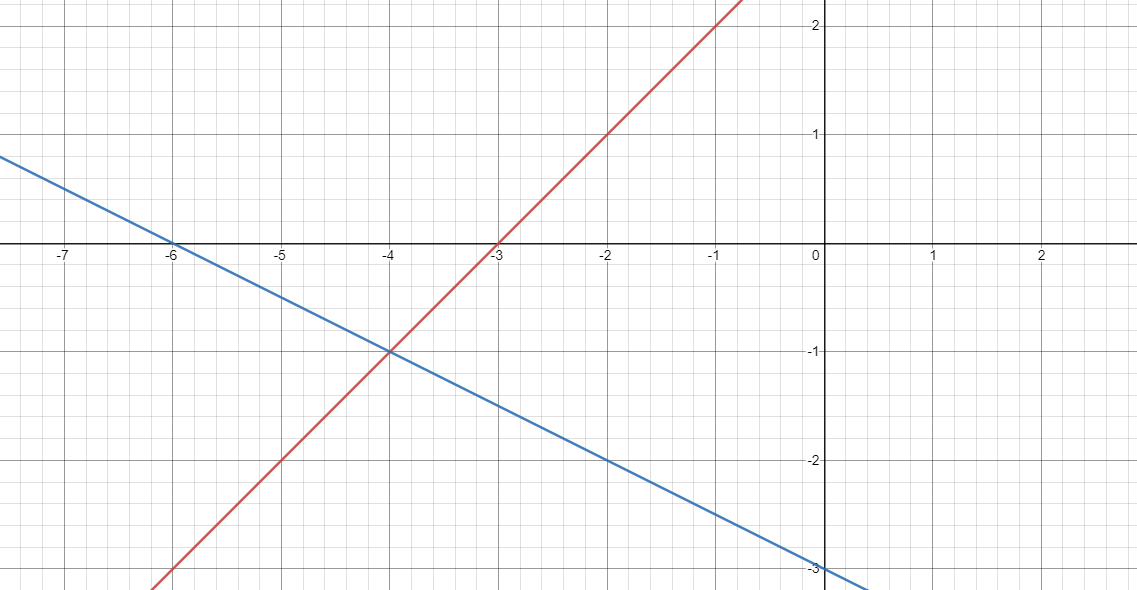### ¿Todavía tienes preguntas de matemáticas?

Pregunte a nuestros tutores expertos
Algebra
PreguntaSolve the following system of equations graphically on the set of axes below. $$y = x + 3$$$$y = - \frac { 1 } { 2 } x - 3$$Plot two lines by clicking the graph. Click a line to delete it.

(-4,-1)